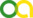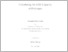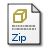Krupp, Stefan (2020). Calculating the EHZ Capacity of Polytopes. PhD thesis, Universität zu Köln.Preview
PDF
DissertationKrupp.pdfZIP
AlgorithmenKrupp.zip

## Abstract

In this thesis we are concerned with symplectic geometry. This rather new field of research gained much interest due to some famous results in the late 20th century, such as the celebrated non-squeezing theorem by Gromov. An intriguing feature of this theorem is, that it is true if and only if there are global symplectic invariants that satisfy certain properties. These global invariants are called symplectic capacities and their existence is a nontrivial fact. By now, several constructions are known but the computation of symplectic capacities has not received much attention. In this thesis, we address this aspect for a certain symplectic capacity, namely the Ekeland-Hofer-Zehnder capacity, which maps a nonnegative number or infinity to every convex body in $\mathbb{R}^{2n}$. To compute the Ekeland-Hofer-Zehnder capacity of sets of the form $K\times T$, where both $K$ and $T$ are convex sets, we use an approach based on Minkowski Billiards. More precisely, we build on an algorithm by Alkoumi and Schlenk, that is formulated for the case where $K$ is a two-dimensional convex set and $T$ is the Euclidean unit ball. On the one hand, we adapt this algorithm to allow convex sets $K$ with arbitrary dimension. On the other hand, we generalize the approach by Alkoumi and Schlenk from the Euclidean setting (where $T$ is the Euclidean unit ball) to the Minkowski setting (where $T$ is an arbitrary convex set). In particular, we consider the case where both $K$ and $T$ are polytopes. Aside from this approach we consider a formulation of the Ekeland-Hofer-Zehnder capacity as a maximization problem, which is due to Abbondandolo and Majer. This maximization problem is a hybrid of a quadratic assignment problem and a quadratic program with non-convex objective function. We employ different optimization techniques and obtain upper and lower bounds on the Ekeland-Hofer-Zehnder capacity of polytopes. Amongst others, we obtain a very good upper bound that is equal to the exact value for many small problem instances at the cost of a rapidly increasing running time.

Item Type: Thesis (PhD thesis)
Translated title:
TitleLanguage
Berechnung der EHZ Kapazität von PolytopenGerman
Translated abstract:
AbstractLanguage
In dieser Arbeit betrachten wir die symplektische Geometrie. Dieser eher junge Forschungsbereich stößt seit dem späten 20. Jahrhundert auf zunehmend Interesse aufgrund einiger namhafter Resultate wie dem berühmten Non-squeezing Theorem von Gromov. Eine Besonderheit dieses Theorems ist die Tatsache, dass seine Aussage genau dann wahr ist, wenn es globale symplektische Invarianten gibt, die bestimmte Eigenschaften erfüllen. Diese globalen Invarianten werden symplektische Kapazitäten genannt und ihre Existenz ist eine nicht triviale Tatsache. Mittlerweile sind mehrere Konstruktionen bekannt, jedoch wurde die Berechnung symplektischer Kapazitäten bislang kaum betrachtet. In dieser Arbeit beschäftigen wir uns mit diesem Gesichtspunkt für eine bestimmte symplektische Kapazität, nämlich der Ekeland-Hofer-Zehnder Kapazität, welche jedem konvexen Körper in $\mathbb{R}^{2n}$ eine nichtnegative Zahl oder $\infty$ zuordnet. Um die Ekeland-Hofer-Zehnder Kapazität von Mengen der Form $K\times T$ zu berechnen, wobei $K$ und $T$ konvexe Mengen sind, verwenden wir einen Ansatz, der auf Minkowski Billards basiert. Genauer bauen wir auf einem Algorithmus von Alkoumi und Schlenk auf, der zweidimensionale konvexe Mengen $K$ betrachtet und annimmt, dass $T$ die euklidische Einheitskugel ist. Einerseits bearbeiten wir diesen Algorithmus so, dass auch konvexe Mengen $K$ mit beliebiger Dimension betrachtet werden können. Andererseits verallgemeinern wir den Ansatz von Alkoumi und Schlenk vom euklidischen Fall (wo $T$ die euklidische Einheitskugel ist) zum Minkowski Fall (wo $T$ eine beliebige konvexe Menge ist). Insbesondere betrachten wir die Situation, in der $K$ und $T$ Polytope sind. Abgesehen von diesem Ansatz betrachten wir ein Resultat von Abbondandolo und Majer, welches die Ekeland-Hofer-Zehnder Kapazität als ein Maximierungsproblem formuliert. Dieses Maximierungsproblem ist ein Hybrid aus einem quadratischen Zuordnungsproblem und einem quadratischen Programm mit nichtkonvexer Zielfunktion. Wir verwenden verschiedene Optimierungstechniken, um obere und untere Schranken an die Ekeland-Hofer-Zehnder Kapazität von Polytopen zu finden. Unter Anderem erhalten wir eine sehr gute obere Schranke, die für viele kleinere Probleminstanzen dem Optimalwert entspricht, jedoch eine schnell wachsende Laufzeit aufweist.German
Creators:
CreatorsEmailORCIDORCID Put Code
Krupp, Stefankrupp@math.uni-koeln.deUNSPECIFIEDUNSPECIFIED
URN: urn:nbn:de:hbz:38-361968
Date: 2020
Language: English
Faculty: Faculty of Mathematics and Natural Sciences
Divisions: Faculty of Mathematics and Natural Sciences > Department of Mathematics and Computer Science > Mathematical Institute
Subjects: Mathematics
Uncontrolled Keywords:
KeywordsLanguage
symplectic geometry; symplectic capacities; billiard dynamics; convex optimization; completely positive optimization; semidefinite optimization; algorithmsEnglish
symplektische Geometrie; symplektische Kapazitäten; Billard Dynamik; konvexe Optimierung; vollständig positive Optimierung; semidefinite Optimierung; AlgorithmenGerman
Date of oral exam: 18 February 2021
Referee:
Vallentin, FrankProf. Dr.
Geiges, HansjörgProf. Ph.D. (Cantab)
Funders: DFG Sonderforschungsbereich CRC/TRR 191, Projekt C1
Projects: C1: Symplectic capacities of polytopes
Refereed: Yes
URI: http://kups.ub.uni-koeln.de/id/eprint/36196View Item